﻿ The Flat Rotation Curve of the Spiral Galaxies may be the Result of the Perfect Combination of the Fluid Mechanics and the Gravitational MechanicsPublications are Open
Access in this journal
Article Versions
Export Article
• Normal Style
• MLA Style
• APA Style
• Chicago Style
Research Article
Open Access Peer-reviewed

### The Flat Rotation Curve of the Spiral Galaxies may be the Result of the Perfect Combination of the Fluid Mechanics and the Gravitational Mechanics

Hongjun Pan
International Journal of Physics. 2019, 7(2), 44-49. DOI: 10.12691/ijp-7-2-2
Received May 16, 2019; Revised July 03, 2019; Accepted July 17, 2019

### Abstract

A new hypothesis is proposed to explain the flat rotation curve of the spiral galaxies. The spiral galaxies can be treated as ideal fluid systems. For Milky Way, the mass density is constant within the radius of 0.65 kpc in the central portion of the bugle and results the linear relationship between the rotation velocity and the distance to the galactic center. The rotation of the spiral galaxies is driven by the central galactic bars and follows both fluid mechanics and gravitational mechanics, the flat rotation curve of the spiral galaxies is the result of the perfect combination of the fluid mechanics and the gravitational mechanics, the nature has balanced itself very well, the mass density distribution indirectly extracted from NASA’s near-infrared image of the Milky Way seems matching the hypothesis. Therefore, the “dark matter” could be unnecessary for the purpose of adding additional gravitational matter, but this does not deny the possible existence of the proposed dark matter in the universe. The feature of the flat rotation curve could be established during the formation of the galaxies from vast clouds of gas and dust, then, is maintained by gravitational force among those celestial objects, gases and interstellar medium, and such gravitational force acts as a binding force and fluid viscosity. After galactic merging, the combination of the fluid mechanics and gravitational mechanics is the only way to restore the galaxies into nice symmetric disk-like morphology with flat feature of the rotation curve after enough evolution time. The super black holes in the centers of the galaxies could be the rotation engines for whole rotation of galaxies. This model may not be perfect and could be improved in the future.

### 1. Introduction

In astronomy and cosmology, accurate rotation curve of spiral galaxies is critical for modeling the mass distribution of the galaxies. The measurement of the orbital rotation velocity is based on the observation of the HII line which gives the rotation velocity limited to the a distance of about up to 4 kpc from the Sun on account of interstellar absorption, and the observation of 21 cm line which gives the rotation velocity in the inner region of Milky Way galaxy 1. In a typical spiral galaxy, the stars and stellar medium circulate around the galactic center, it is expected that the circulation would follow Kepler's laws; however, based on the observed data, the rotation curve of Milky Way galaxy is relatively flat when the distance to the center is greater than 2.25 kpc, i.e., the average rotation speed is about 213 km/s 2. The flat rotation curve of galaxy M33 is also observed when the distance is beyond 3 kpc 3, such flat pattern of the rotation curve are very common in spiral galaxies. Persic et. al. studied the feature of the rotation curve from 1100 optical and radio rotation curves and relative surface photometry and derived the equation of the universal rotation curve based the luminosity 4. Karukes and salucci also studied the universal rotation curves of dwarf disc galaxies 5. So far, there is no reasonable explanation for the flat rotation curve based on the estimated mass, the new concept of dark matter was introduced to add more gravitational mass 6, 7, great efforts and resources have been put to search the possible dark matter, so far no success. In this paper, a new hypothesis is proposed to explain the flat feature of the rotation curve based on the fluid mechanics and the gravitational mechanics, which may shed new light to understand the spiral galaxies.

### 2. Rotation of Ideal Fluid in the Space

Let us “perform” an experiment with an ideal fluid in the outer space where there is no gravity effect and think about the reasonable result based the basic knowledge of the fluid. Assume that the ideal fluid has the right viscosity and the right surface tension. In the outer space, the shape of the fluid will be spherical without a holding container, a rigid bar spinner with length L is placed in the center of the fluid ball shown in Figure 1. When the bar spinner starts to rotate around its central axis, it will drive the fluid near it to rotate also, such rotation will spread to all fluid of the ball due to the viscosity, the centrifuge force in the rotation plane will push the fluid outwards in the rotation plane. When intermolecular interaction force and the surface tension are greater or enough to balance the rotation centrifuge force at a proper rotation speed, the rotation becomes stable at the ideal rotation speed, the shape of the fluid will look like (or very close to) a discus. Figure 2 A is the view of the rotating fluid from above the rotation plane and Figure 2 B is the cross section view of the fluid shape from side of the fluid (in the rotation plane).

• Figure 1. An ideal fluid ball with a bar-like spinner in the center is placed in the space without gravity
• Figure 2 A. Above rotation plane view of rotation fluid
• Figure 2. B. Cross section side view of rotation fluid

All fluid beyond the rotation radius r0 (the half of its length L) of the spinner will have the same rotation velocity as the edge r0 of the spinner; the rotation velocity of the fluid within r0 range (especially in the blue regions near the spinner bar in Figure 2A) will linearly increase with the distance from the center. The expected rotation curve of the fluid in this case is illustrated (or very close) in Figure 3 (not the real test data), the R is the radius of the fluid discus. Actually, such experiment can be conducted in the space station with proper fluid for verification.

• Figure 3. Rotation curve of ideal fluid in the space (illustration only, not the real test data)

If additional fluid material is added to a non-central position of the stable rotating fluid discus, the symmetrical distributed discus, the symmetry of fluid distribution and the rotation curve of Figure 3 will be destroyed. However, the symmetrical distribution of the fluid and the rotation curve of Figure 3 will be restored after enough time rotation.

### 3. The Rotation Curves of Spiral Galaxies

• Figure 4. Corrected rotation curve of Milky Way based on the observed data and correction. Credit: Laurent Chemin et al. 
• Figure 5. The M33 rotation curve, Credit: Edvige Corbelli and Paolo Salucci 

Figure 4 is the rotation curve (green open circles) of Milky Way produced by Chemin, et. al. based on the observed data with correction 2. Figure 5 is the rotation curve of M33 by Corbelli, E.; Salucci, P. 3. Please note that other lines in the original figures are digitally removed for simplicity. In the Figure 4, it is only plotted up to 8 kpc, however, other observed data shows that the rotation curve beyond 8 kpc is also flat 8.

We can see in both curves that the rotation velocity linearly and quickly increases at beginning short distance from the galactic center (about 0.65 kpc in Milky Way and 1.5 kpc for M33), the data for this linear section was taken near bar area which is similar to the blue regions in Figure 2A. The velocity quickly levels off and becomes flat. Since we reside inside of Milky Way, the measurement of the rotation velocity of the Milky Way should be much more accurate than those data taken from other remote spiral galaxies due to the possible systematical measurement error in such cosmic scale distance. Such rotation curves are very similar to the expected rotation curve in Figure 3. Therefore, the central portion of the galactic bar can be fairly treated as a “rigid” object and the rotation of the whole spiral system can be treated as ideal fluid rotation system driven by the rotation of the galactic bar. However, the situation of the spiral galaxies is still significantly different from the ideal rotating fluid disc which is driven by rigid rotation bar spinner. The typical universal rotation curve can be drawn in Figure 6.

• Figure 6. A typical universal rotation curve of spiral galaxy

The rotation curve of Figure 6 can be divided into 3 sections:

Section 1: from 0 to r1, the rotation velocity linearly increases with the distance from the galactic center.

Section 2: from r1 to r2, the rotation velocity gradually flats off from linearity relationship.

Section 3: from r2 to the edge R of the galactic disc, the rotation velocity is constant.

Each section will be addressed in detail for the Milky Way galaxy.

### 4. Mass distribution of Milky Way

4.1. Mass Distribution in Section 1

From the Figure 4, the linear rotation curve of Milky Way is from 0 to radius 0.65 kpc. This is located at the central area of the galaxy with 3-dimentional nuclear bulge in the center. It is assumed that at least at current time, the Milky Way is relatively stable, all forces related to the rotation have to be balanced. Based on the Newtonian physics, for the circular motion, the centripetal force must equal to the gravitational force, so the mass within the orbital rotation radius r is:(1)

Where, M(r) is the total mass inside the orbital radius r,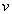is the orbital velocity and G is the gravitational constant. The velocity follows the equation:(2)

Whereis a proportional constant.

Put the equation (2) into (1):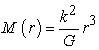(3)

The central area of the bulge can be treated approximately as spherical shape, the volume of a sphere with radius r is:(4)

The equation (3) can be further written as: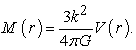(5)

Because the mass is equal to the mass density times the volume, the mass density ρ(r) is: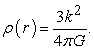(6)

So, the mass density in this region is constant, does not change with the radius, such result is based on the observation only without any assumption, this is very interesting. Many people used decay mass distribution function for the central area of the spiral galaxies 9, 10, 11, the linear rotation curve for this central area reveals different mass distribution.

For Milky Way, the parameterextracted from Figure 4 is:(7)

The mass density in the central area (r ≤ 0.65 kpc) is: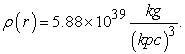(8)

The total mass within radius r = 0.65 kpc with spherical volume of 1.15 (kpc)3 is(9)

Portail et. al. studied in detail about the Milky Way box/peanut bugle structure and calculated the total mass of the bulge 11, they found that within ellipsoid bulge sizethe total mass is 1.84Mʘ, where, Mʘ is the solar mass which is equal to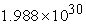kg. So the total mass of the bulge within the ellipsoid volume of 15.48 (kpc)3 is: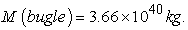(10)

The mass ratio of (9) to the (10) is 0.18 or 18%, but the volume ratio is about 0.074 or 7.4%, so the 7.4% of bulge central volume holds 18% of total bulge mass, this reflects that the central area of the bulge is the core area with very high mass density. This agrees with general expectation that the center of the bulge has the highest mass density.

For M33 galaxy, based on the Figure 5, the parameter k in the equation (2) within the linear range is: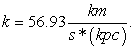(11)

And the mass density in the central area is: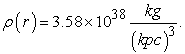(12)

However, it should be understood that it is the 3-dimentional constant mass distribution which causes the linear relationship between the rotation velocity and the distance to the center of galaxy, acting like “rigid” rotating bar.

4.2. Mass Distribution in Section 2

In this section from 0.65 kpc to 2.25 kpc, the mass distribution gradually transits from 3-dimentional spherical to 2-dimentional disc-like distribution, it is difficult to use a well defined physical equation to model the mass distribution. It can be nicely fit by the following mathematic equation: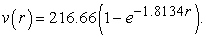(13)

The total mass within the orbital rotation radius r still follows the equation (1). There is no such transition period in Figure 3.

4.3. Mass Distribution in Section 3

In this section of rotation curve, the velocity of the objects with orbital rotation radius greater than r2 (2.25 kpc for Milky Way) is roughly constant, the average rotation velocity is:(14)

so the total mass density at a circle with radius r is:(15)

Where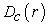is the circular mass density at the circle with radius r. Since the velocityis constant, this means that the total circular mass density is constant, the mass density along the radius r is:(16)

Where,is the linear mass distribution along the radius r. For Milky Way galaxy, the orbital velocity of the Sun is 213 km/s (based on the Figure 4), which represents the average orbital velocity of the galaxy with orbital radius greater than 2.25 kpc (based on Figure 4), then,(17)

Figure 7 is the near-infrared image of Milky Way from observations made by the COBE satellite 12. The mass distribution of the Milky Way galaxy beyond the central area can be viewed as 2-dimentional distribution along the galactic disc plane. We can roughly believe that the luminosity more or less represents the density of the mass because the brighter the higher the star density is (this could be oversimplified). It is interesting that image profile at the central area is relatively flat, this may be due to the X-structure in the galactic center, and the left side is slight bigger than right side, this is most likely due to projection effect. The luminosity gradually decreases beyond the central area. This image can be converted to bitmap format, so the luminosity can be represented by only one number. Such bmp image can be further converted to XYZ data file, in which, the X is the horizontal pixel value (i.e., the radius of the galaxy), Y is the vertical pixel value and the Z is luminosity value at (X, Y) location. The integration of all Z values along Y at each X will represent the total mass density at the X (or the radius r).

Figure 8 is the BMP image (bottom) of Figure 7; the white line (above) is the integration of luminosity along the Y which represents the mass density distribution along the radius of Milky Way. One can see that the mass density at the central area is relatively flat and quickly decreases in the transition range. The mass density gradually decreases with the increase of the radius beyond the transition area. The data fitting lines with equation (16) are shown with blue and red curves for the left and right side of the image, respectively; and the fitting formula with parameters are shown in the image. One can see that the data can be well fit by the equation (16). The fitting parameters of left side and right side are slightly different which could be also the projection effect, so the fitting formulas for the data range rwith average parameters is: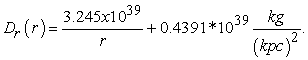(18)

The 0.4391 x 1039 is the background radiation.

• Figure 7. A near-infrared image of Milky Way from observations made by the COBE satellite. Credit: NASA website
• Figure 8. The BMP image (bottom) of Figure 6, and the mass density distribution along galactic radius with fitting (above)

The red line in Figure 4 is the fitting line with equations (2) (13) and (14).

### 5. Discussion

It seems that the proposed hypothesis explains the observed rotation curves of spiral galaxies well and the data from the Milky Way image matches the model well, the central portion of the galactic bar can be viewed as a “rigid” item in rotation and the whole rotation of the galaxies is driven by the rotation of the “rigid” bar and can be treated as an ideal fluid system, the rotation of the spiral galaxies follows the universal gravitational laws. This means that the “dark matter” for the purpose of additional gravitational force may be unnecessary, the nature has balanced itself very well. However, this does not deny the possible existence of proposed dark matter in the universe. The concept of dark energy was also proposed to explain the expansion of the universe. Such proposed dark matter and dark energy are among the most important research subjects in modern cosmology, the possible fluid property of the dark matter and dark energy has been extensively studied also 13, 14, 15, 16, 17. The feature of the flat rotation curve could be established during the formation of the galaxies from vast clouds of gas and dust, then, is maintained by gravitational force among those celestial objects, gases and interstellar medium, such gravitational force acts as a binding force and fluid viscosity. Many strong evidences show that a super massive black hole is located at the center of the our Milky Way galaxy 18, 19 near the Sgr A. It is commonly believed that all spiral galaxies have a supper massive black hole in the center of the galaxies. Most likely, the rotation of the galactic bars are controlled by the super massive black holes, the angular rotation speed of the galactic bars reflects the angular rotation speed of the black holes. Therefore, the rotation of the whole galaxies are driven by the central black holes.

It is estimated that the number of galaxies in the universe may be up to 2 trillion 20. Galactic collisions and mergers are very common events in the universe. Our Milky Way could have up to 18 mergers in the history 21. Each such collision/merging will cause huge chaos in the mass distribution and the original symmetry of the mass distribution will be terribly destroyed as the merging example shown in Figure 9, where the Antennae Galaxies NGC 4038 and NGC 4039 are merging, some portions of the original spiral arms can still be seen, but the arms and the symmetry of the merging galaxies are terribly destroyed. Our Milky Way galaxy currently has very symmetrical disk-like morphology and mass distribution, if the galaxy mergers did happen in its history, the combination of the ideal fluid rotation mechanics and the gravitational mechanics should be the only way to restore it to its current morphology with the flat feature of the rotation curve after enough time evolution.

• Figure 9. Merging of NGC 4038 & 4039. Credit: NASA website 

### 6. Conclusion

The spiral galaxies can be treated as ideal fluid systems with the central portion of the galactic bars acting as “rigid” bars due to 3-dimentional spherical mass distribution with constant mass density. The rotation of the spiral galaxies is driven by the central galactic bars and follows both fluid mechanics and gravitational mechanics, the flat rotation curve of the spiral galaxies is the result of the perfect combination of the fluid mechanics and the gravitational mechanics, the radial mass density distribution indirectly extracted from NASA’s near-infrared image seems matching the hypothesis. Therefore, the “dark matter” could be unnecessary for purpose of adding more gravitational matter, the nature has balanced itself nicely. This does not deny the possible existence of the proposed dark matter in the universe. The feature of the flat rotation curve could be established during the formation of the galaxies from vast clouds of gas and dust, then, is maintained by gravitational force among those celestial objects, gases and interstellar medium. After galactic merging, the combination of the fluid mechanics and gravitational mechanics is the only way to restore the galaxies into nice symmetric disk-like morphology with flat feature of the rotation curve after enough evolution time. The super black holes in the centers of the galaxies could be the rotation engines for whole rotation of galaxies. This model may not be perfect and could be improved in the future.

### References

  Lequeux, J. Structure and Evolution of Galaxies, pp 27. Gordon and Breach science publishers. 1969. In article  Chemin, L., Renaud F., & Soubiran, C. “The incorrect rotation curve of the Milky Way”, Astron Astrophys. 578, A14, 2015. In article View Article  Corbelli, E., Salucci, P. “The extended rotation curve and the dark matter halo of M33”, MNRAS, 311 (2), pp 441-447, 2000. In article View Article  Massimo Persic Paolo Salucci Fulvio Stel. The universal rotation curve of spiral galaxies — I. The dark matter connection MNRAS, 281 (1), pp 26-47, 1996. In article View Article  Karukes, E, Salucci, P. The universal rotation curve of dwarf disc galaxies, MNRAS 465, 4703-4722. (2017). In article View Article  Koupelis, T., Kuhn, K. In Quest of the Universe. pp. 492, Figure 16-13, Jones & Bartlett Publishers, 2007. In article  Jones, M., Lambourne, R. & Adams, D. An Introduction to Galaxies and Cosmology, pp 21, Figure 1.13, Cambridge University Press, 2004. In article  Mroz, P., Udalski, A., Skowron, D. et al. “Rotation curve of the Milky Way from Classical Cepheids”. ApJL, 870:L10, 2018. In article View Article  Dwek, E., Arendt, R., Hauser, M., et. al., “Morphology, Near-infrared Luminosity, and Mass of the Galactic Bugle from COBE DIRBE Observation”, ApJ., 445, pp 716-730, 1995. In article View Article  Cao, L., Mao, S., Tataf, D., Rattenbury, N., “A New Photometric Model of the Galactic Bar Using Red Clump Giants”, MNRAS 434, pp 595-605, 2013. In article View Article  Portail, M., Wegg, C., Gerhard, O., Martinez-Valpuesta, I., “Made-to Measure Models of the Galactic Box/Peanut Bugle: Stellar and Total Mass in the Bugle Region”, MNRAS 448, pp 713-731, 2015. In article View Article  [Online]. Available: https://imagine.gsfc.nasa.gov/science/objects/milkyway1.html. [Accessed July 12, 2019]. In article  Farnes, J. “A Unifying Theory of Dark Energy and Dark Matter: Negative Masses and Matter Creation within a Modified ΛCDM Framework”, A & A 620, A92. 2018. In article View Article  Arbey, A., “Is It Possible to Consider Dark Energy and Dark Matter as a Same and Unique Dark Fluid?”, arXiv:astro-ph/0506732, 2005. In article  Arbey, A., "Dark Fluid: a Complex Scalar Field to Unify Dark Energy and Dark Matter". Phys. Rev. D., 74 (4): 043516, 2006. In article View Article  Pan, H., “‎Application of Fluid Mechanics to Dark Matter”, Int. J. Mod. Phys. 20(14), 3135-3137. 2005. In article View Article  Pan. H., “The Redshift and Geometrical Aspect of Photons”, arXiv:0704. 1044, 2007. In article  Ghez, A., Salim, S., Weiberg, N. et. al. “Measuring Distance and Properties of the Milky Way's Central Supermassive Black Hole with Stellar Orbits”, ApJ. 689, pp 1044-1062, 2008. In article View Article  Abuter, R., Amorim, R., Baubock, M. et al. (2019), “A Geometric Distance Measurement to the Galactic Center Black Hole with 0.3% Uncertainty”, Astron. Astrophys. 625, L10, 2019. In article View Article  Conselice, C., Wilkinson, A., Duncan, K., Mortlock, A., “The Evolution of Galaxy Number Density at z < 8 and Its Implications”, ApJ, 830:83, 2016. In article View Article  Kruijssen, J., Pfeffer, J., Reina-Campos, M., Crain, R., Bastian, N., “The Formation and Assembly History of the Milky Way Revealed by Its Globular Cluster Population”, MNRAS 486, pp 3180-3202, 2019. In article View Article  [Online] Available: https://apod.nasa.gov/apod/ap120812.html. [Accessed July 12, 2019]. In articleThis work is licensed under a Creative Commons Attribution 4.0 International License. To view a copy of this license, visit http://creativecommons.org/licenses/by/4.0/